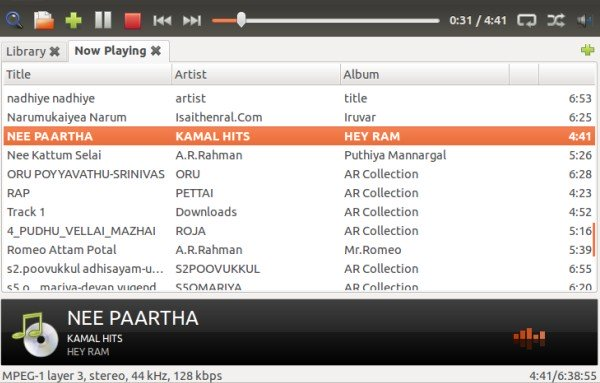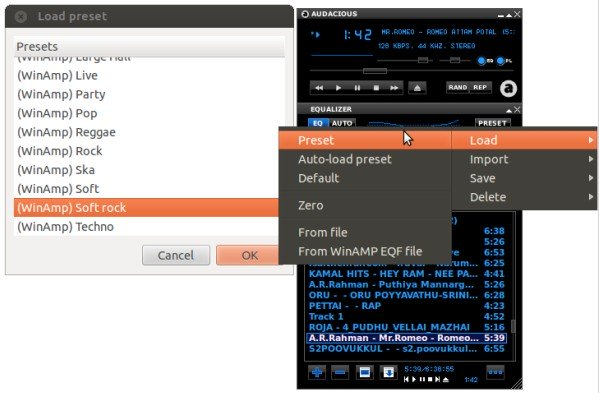# How to install audacious in ubuntu 12.04

Audacious is a light weight mp3 player which uses less resources and gives high quality audio . It comes with two interfaces ( GTK interface and Winamp interface ) . Winamp interface looks like old winamp 2.x version has a same functionality like playlist,presets etc..In this article we will see about Audacious installation in ubuntu 12.04 and importing presets.

# Installing audacious in ubuntu 12.04 :

Audacious installation in ubuntu 12.04 is pretty easy , just issue the below three commands one by one.
» Add the repository using below command .
`krizna@leela:~\$ sudo add-apt-repository ppa:nilarimogard/webupd8 `
» Update the repository
`krizna@leela:~\$ sudo apt-get update `
» Issue the below command to install audacious.
`krizna@leela:~\$ sudo apt-get install audacious`
That’s it ..By default it has GTK interface .## Winamp interface

If you want winamp interface . just Goto View -> Interface and choose winamp classic interface

## Import presets

Presets are not available by default, you will have to add yourself . follow the steps to add presets.

» Unzip and copy eq.preset file to /home/username/.config/audacious/  ( if already there , just replace it )
`krizna@leela:~\$ cp eq.preset .config/audacious/`

» Restart audacious and click preset , now you can choose your favourite preset.Enjoy 🙂

• quatra

After 2 days of trying and frustration I finally got it to work without downloading anything. I upgraded my Audacious with the nilarimogard/webupd8 repository to version 3.4 and then pasting the following into a terminal:

rm \$HOME/.config/audacious/eq.preset
cat > \$HOME/.config/audacious/eq.preset << EOF

[Presets]
Preset0=Classical
Preset1=Club
Preset2=Dance
Preset3=Flat
Preset4=Live
Preset6=Rock
Preset7=Pop
Preset8=Full Bass and Treble
Preset9=Full Bass
Preset10=Full Treble
Preset11=Soft
Preset12=Party
Preset13=Ska
Preset14=Soft Rock
Preset15=Large Hall
Preset16=Reggae
Preset17=Techno

[Classical]
Preamp=0.375
Band0=0.375
Band1=0.375
Band2=0.375
Band3=0.375
Band4=0.375
Band5=0.375
Band6=-4.5
Band7=-4.5
Band8=-4.5
Band9=-6

[Club]
Preamp=0.375
Band0=0.375
Band1=0.375
Band2=2.25
Band3=3.75
Band4=3.75
Band5=3.75
Band6=2.25
Band7=0.375
Band8=0.375
Band9=0.375

[Dance]
Preamp=0.375
Band0=6
Band1=4.5
Band2=1.5
Band3=0
Band4=0
Band5=-3.75
Band6=-4.5
Band7=-4.5
Band8=0
Band9=0

[Flat]
Preamp=0.375
Band0=0.375
Band1=0.375
Band2=0.375
Band3=0.375
Band4=0.375
Band5=0.375
Band6=0.375
Band7=0.375
Band8=0.375
Band9=0.375

[Live]
Preamp=0.375
Band0=-3
Band1=0.375
Band2=2.625
Band3=3.375
Band4=3.75
Band5=3.75
Band6=2.625
Band7=1.875
Band8=1.875
Band9=1.5

Preamp=0.375
Band0=3
Band1=6.75
Band2=3.375
Band3=-2.25
Band4=-1.5
Band5=1.125
Band6=3
Band7=6
Band8=7.875
Band9=9

[Rock]
Preamp=0.375
Band0=4.875
Band1=3
Band2=-3.375
Band3=-4.875
Band4=-2.25
Band5=2.625
Band6=5.625
Band7=6.75
Band8=6.75
Band9=6.75

[Pop]
Preamp=0.375
Band0=-1.125
Band1=3
Band2=4.5
Band3=4.875
Band4=3.375
Band5=-0.75
Band6=-1.5
Band7=-1.5
Band8=-1.125
Band9=-1.125

[Full Bass and Treble]
Preamp=0.375
Band0=4.5
Band1=3.75
Band2=0.375
Band3=-4.5
Band4=-3
Band5=1.125
Band6=5.25
Band7=6.75
Band8=7.5
Band9=7.5

[Full Bass]
Preamp=0.375
Band0=6
Band1=6
Band2=6
Band3=3.75
Band4=1.125
Band5=-2.625
Band6=-5.25
Band7=-6.375
Band8=-6.75
Band9=-6.75

[Full Treble]
Preamp=0.375
Band0=-6
Band1=-6
Band2=-6
Band3=-2.625
Band4=1.875
Band5=6.75
Band6=9.75
Band7=9.75
Band8=9.75
Band9=10.5

[Soft]
Preamp=0.375
Band0=3
Band1=1.125
Band2=-0.75
Band3=-1.5
Band4=-0.75
Band5=2.625
Band6=5.25
Band7=6
Band8=6.75
Band9=7.5

[Party]
Preamp=0.375
Band0=4.5
Band1=4.5
Band2=0.375
Band3=0.375
Band4=0.375
Band5=0.375
Band6=0.375
Band7=0.375
Band8=4.5
Band9=4.5

[Ska]
Preamp=0.375
Band0=-1.5
Band1=-3
Band2=-2.625
Band3=-0.375
Band4=2.625
Band5=3.75
Band6=5.625
Band7=6
Band8=6.75
Band9=6

[Soft Rock]
Preamp=0.375
Band0=2.625
Band1=2.625
Band2=1.5
Band3=-0.375
Band4=-2.625
Band5=-3.375
Band6=-2.25
Band7=-0.375
Band8=1.875
Band9=5.625

[Large Hall]
Preamp=0.375
Band0=6.375
Band1=6.375
Band2=3.75
Band3=3.75
Band4=0.375
Band5=-3
Band6=-3
Band7=-3
Band8=0.375
Band9=0.375

[Reggae]
Preamp=0.375
Band0=0.375
Band1=0.375
Band2=-0.375
Band3=-3.75
Band4=0.375
Band5=4.125
Band6=4.125
Band7=0.375
Band8=0.375
Band9=0.375

[Techno]
Preamp=0.375
Band0=4.875
Band1=3.75
Band2=0.375
Band3=-3.375
Band4=-3
Band5=0.375
Band6=4.875
Band7=6
Band8=6
Band9=5.625
EOF

Nothing to download and it works for me!

I haven't tried it with other Audacious distros but I got the one from the Xubuntu 12.04 LTS Software Center on my laptop and will try tomorrow.

• quatra

After 2 days of trying and frustration I finally got it to work without downloading anything. I upgraded my Audacious with the nilarimogard/webupd8 repository to version 3.4 and then pasting the following into a terminal:

rm \$HOME/.config/audacious/eq.preset
cat > \$HOME/.config/audacious/eq.preset << EOF

[Presets]
Preset0=Classical
Preset1=Club
Preset2=Dance
Preset3=Flat
Preset4=Live
Preset6=Rock
Preset7=Pop
Preset8=Full Bass and Treble
Preset9=Full Bass
Preset10=Full Treble
Preset11=Soft
Preset12=Party
Preset13=Ska
Preset14=Soft Rock
Preset15=Large Hall
Preset16=Reggae
Preset17=Techno

[Classical]
Preamp=0.375
Band0=0.375
Band1=0.375
Band2=0.375
Band3=0.375
Band4=0.375
Band5=0.375
Band6=-4.5
Band7=-4.5
Band8=-4.5
Band9=-6

[Club]
Preamp=0.375
Band0=0.375
Band1=0.375
Band2=2.25
Band3=3.75
Band4=3.75
Band5=3.75
Band6=2.25
Band7=0.375
Band8=0.375
Band9=0.375

[Dance]
Preamp=0.375
Band0=6
Band1=4.5
Band2=1.5
Band3=0
Band4=0
Band5=-3.75
Band6=-4.5
Band7=-4.5
Band8=0
Band9=0

[Flat]
Preamp=0.375
Band0=0.375
Band1=0.375
Band2=0.375
Band3=0.375
Band4=0.375
Band5=0.375
Band6=0.375
Band7=0.375
Band8=0.375
Band9=0.375

[Live]
Preamp=0.375
Band0=-3
Band1=0.375
Band2=2.625
Band3=3.375
Band4=3.75
Band5=3.75
Band6=2.625
Band7=1.875
Band8=1.875
Band9=1.5

Preamp=0.375
Band0=3
Band1=6.75
Band2=3.375
Band3=-2.25
Band4=-1.5
Band5=1.125
Band6=3
Band7=6
Band8=7.875
Band9=9

[Rock]
Preamp=0.375
Band0=4.875
Band1=3
Band2=-3.375
Band3=-4.875
Band4=-2.25
Band5=2.625
Band6=5.625
Band7=6.75
Band8=6.75
Band9=6.75

[Pop]
Preamp=0.375
Band0=-1.125
Band1=3
Band2=4.5
Band3=4.875
Band4=3.375
Band5=-0.75
Band6=-1.5
Band7=-1.5
Band8=-1.125
Band9=-1.125

[Full Bass and Treble]
Preamp=0.375
Band0=4.5
Band1=3.75
Band2=0.375
Band3=-4.5
Band4=-3
Band5=1.125
Band6=5.25
Band7=6.75
Band8=7.5
Band9=7.5

[Full Bass]
Preamp=0.375
Band0=6
Band1=6
Band2=6
Band3=3.75
Band4=1.125
Band5=-2.625
Band6=-5.25
Band7=-6.375
Band8=-6.75
Band9=-6.75

[Full Treble]
Preamp=0.375
Band0=-6
Band1=-6
Band2=-6
Band3=-2.625
Band4=1.875
Band5=6.75
Band6=9.75
Band7=9.75
Band8=9.75
Band9=10.5

[Soft]
Preamp=0.375
Band0=3
Band1=1.125
Band2=-0.75
Band3=-1.5
Band4=-0.75
Band5=2.625
Band6=5.25
Band7=6
Band8=6.75
Band9=7.5

[Party]
Preamp=0.375
Band0=4.5
Band1=4.5
Band2=0.375
Band3=0.375
Band4=0.375
Band5=0.375
Band6=0.375
Band7=0.375
Band8=4.5
Band9=4.5

[Ska]
Preamp=0.375
Band0=-1.5
Band1=-3
Band2=-2.625
Band3=-0.375
Band4=2.625
Band5=3.75
Band6=5.625
Band7=6
Band8=6.75
Band9=6

[Soft Rock]
Preamp=0.375
Band0=2.625
Band1=2.625
Band2=1.5
Band3=-0.375
Band4=-2.625
Band5=-3.375
Band6=-2.25
Band7=-0.375
Band8=1.875
Band9=5.625

[Large Hall]
Preamp=0.375
Band0=6.375
Band1=6.375
Band2=3.75
Band3=3.75
Band4=0.375
Band5=-3
Band6=-3
Band7=-3
Band8=0.375
Band9=0.375

[Reggae]
Preamp=0.375
Band0=0.375
Band1=0.375
Band2=-0.375
Band3=-3.75
Band4=0.375
Band5=4.125
Band6=4.125
Band7=0.375
Band8=0.375
Band9=0.375

[Techno]
Preamp=0.375
Band0=4.875
Band1=3.75
Band2=0.375
Band3=-3.375
Band4=-3
Band5=0.375
Band6=4.875
Band7=6
Band8=6
Band9=5.625
EOF

Nothing to download and it works for me!

I haven't tried it with other Audacious distros but I got the one from the Xubuntu 12.04 LTS Software Center on my laptop and will try tomorrow.